# Problem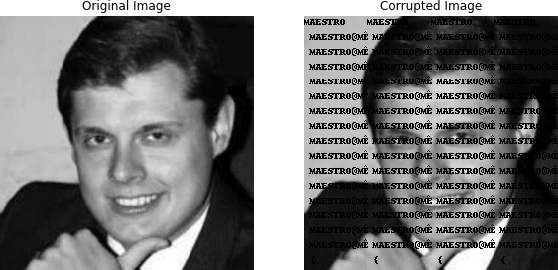## Grayscale image

A grayscale image is represented as an $m \times n$ matrix of intensities $U^{orig}$ (typically between the values $0$ and $255$). We are given all the values of corrupted picture, but some of them should be preserved as is through the recovering procedure: $U^{corr}_{ij} \; \forall (i,j)\in K$, where $K\subset\{1,\ldots,m\}×\{1,\ldots,n\}$ is the set of indices corresponding to known pixel values. Our job is to in-paint the image by guessing the missing pixel values, i.e., those with indices not in $K$. The reconstructed image will be represented by $U \in \mathbb{R}^{m \times n}$, where $U$ matches the known pixels, i.e., $U_{ij}=U^{corr}_{ij}$ for $(i,j)\in K$.

The reconstruction $U$ is found by minimizing the total variation of $U$, subject to matching the known pixel values. We will use the $l_{2}$ total variation, defined as

So, the final optimization problem will be written as follows:

The crucial thing about this problem is defining set of known pixels $K$. There are some heuristics: for example, we could state, that each pixel with color similar (or exactly equal) to the color of text is unknown. The results for such approach are presented below: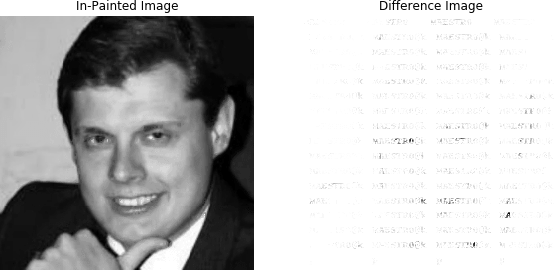## Color image

For the color case we consider in-painting problem in a slightly different setting: destroying some random part of all pixels. In this case the image itself is 3d tensor (we convert all others color chemes to the RGB). As it was in the grayscale case, we construct the mask $K$ of known pixels for all color channels uniformly, based on the principle of similarity of particular 3d pixel to the vector $[0, 0, 0]$ (black pixel). The results are quite promising - note, that we have no information about the original picture, but assumption, that corrupted pixels are black. For the color picture we just sum all tv’s on the each channel:

Then, we need to write down optimization problem to be solved:

Results are presented below (these computations are really take time):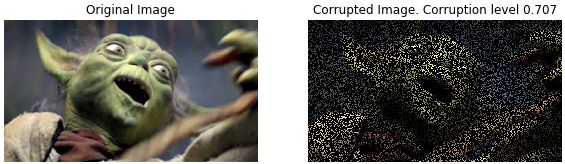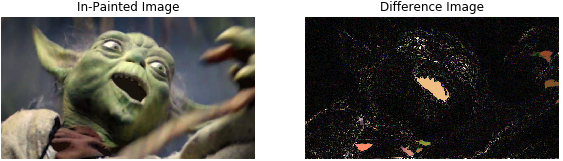It is not that easy, right?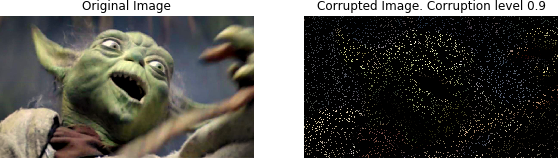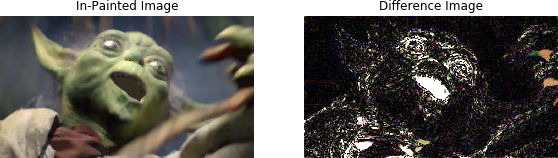Only 5% of all pixels are left: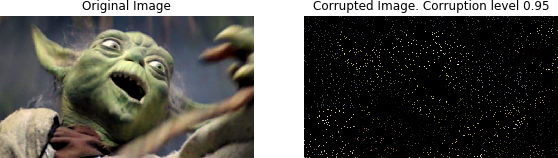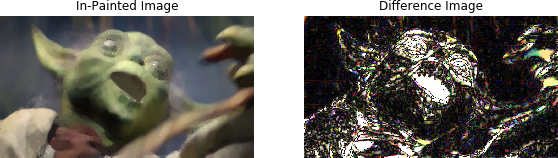What about 1% of all pixels?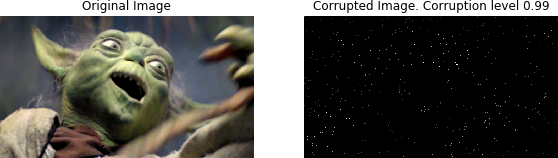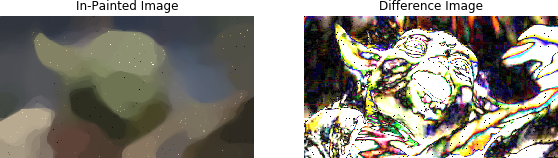# Code